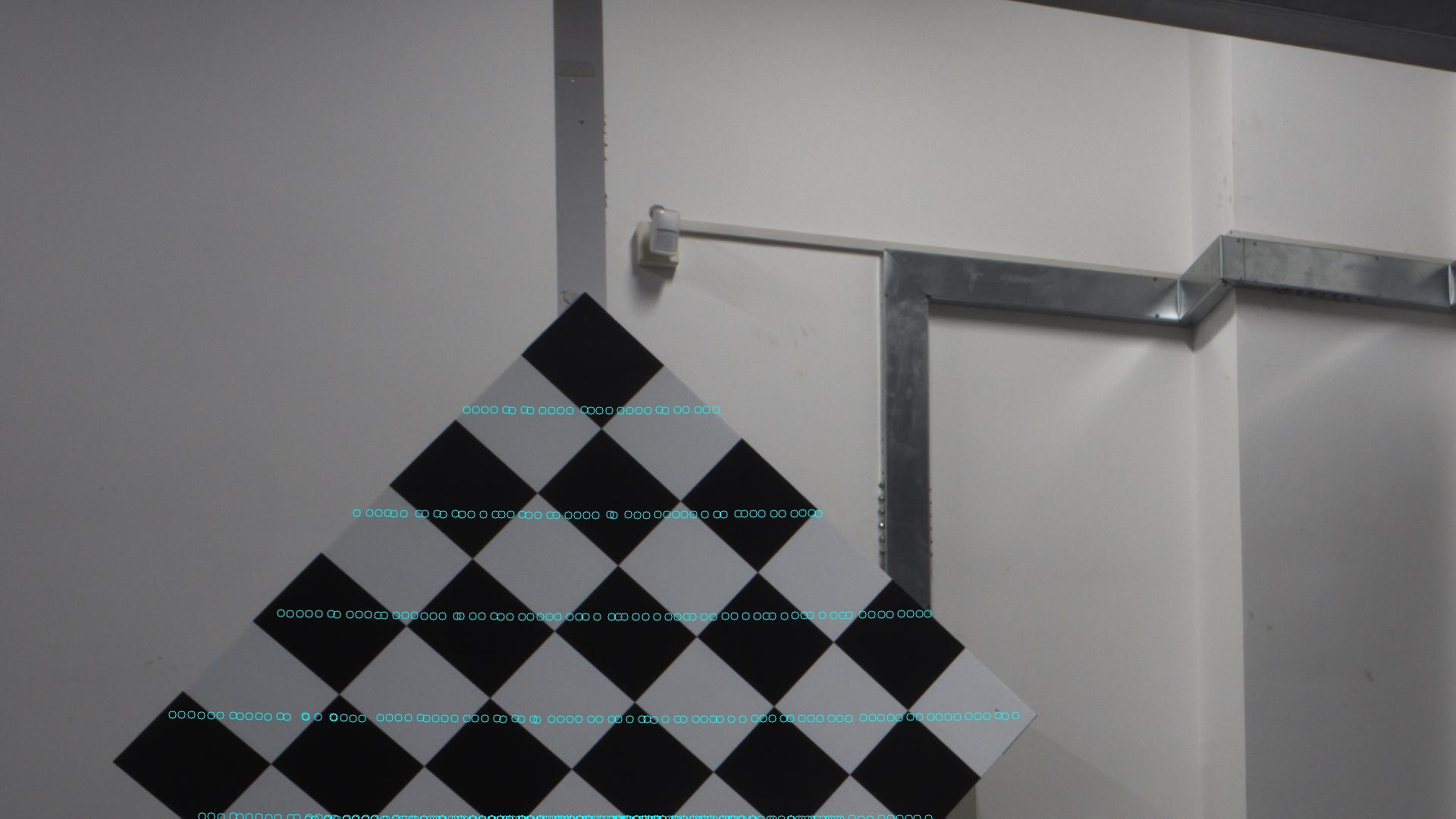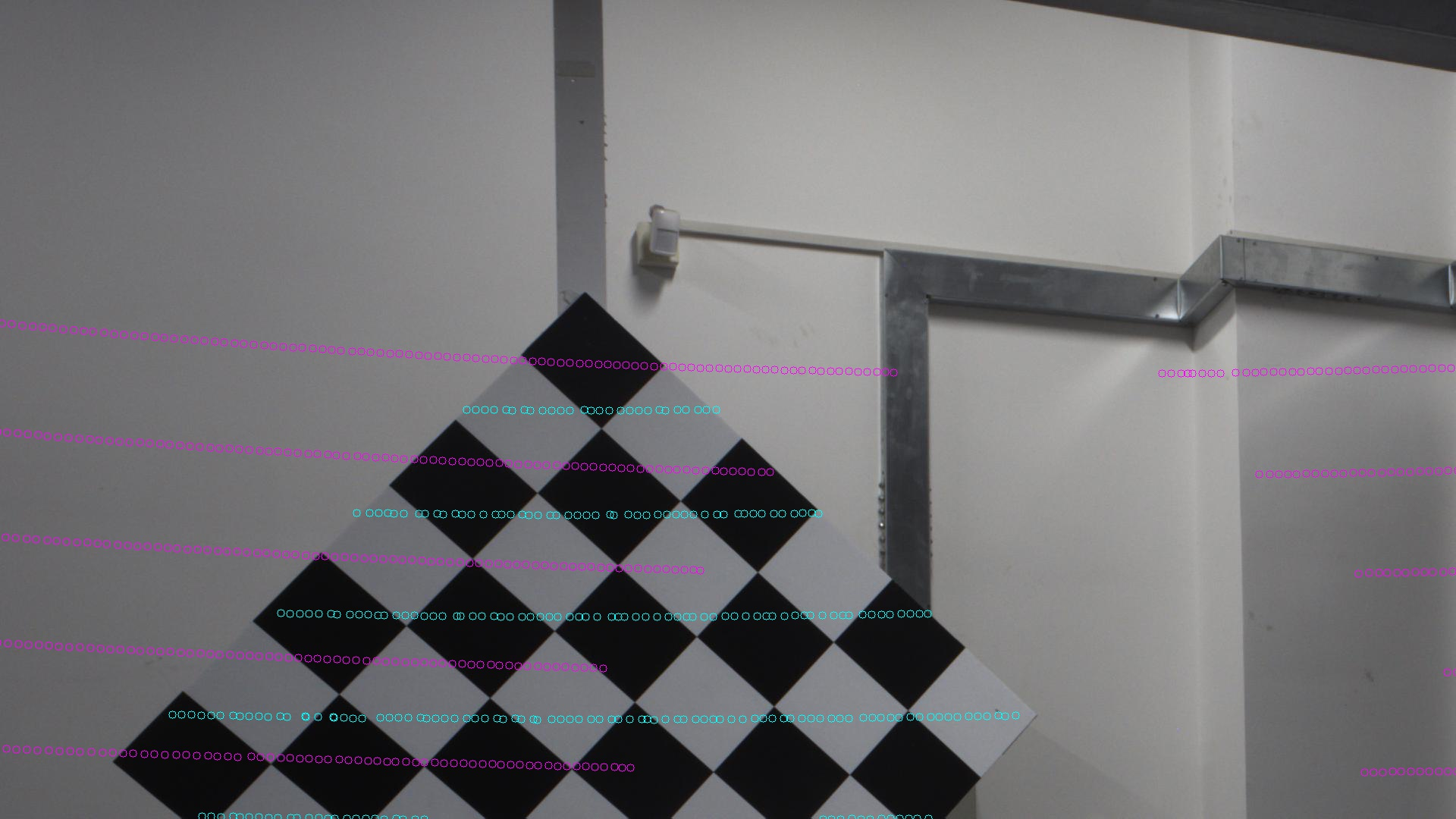# 相机和雷达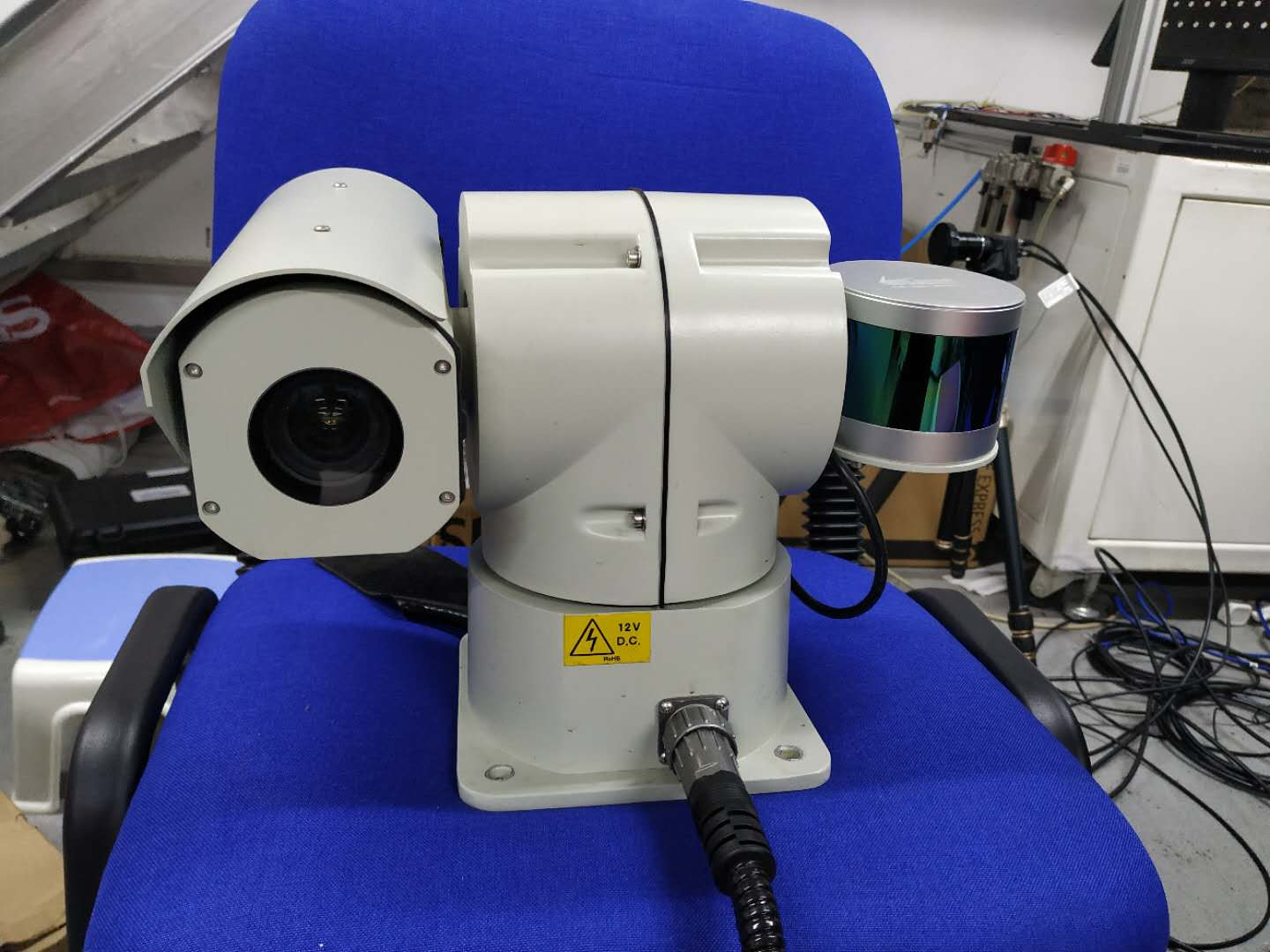# 准备工作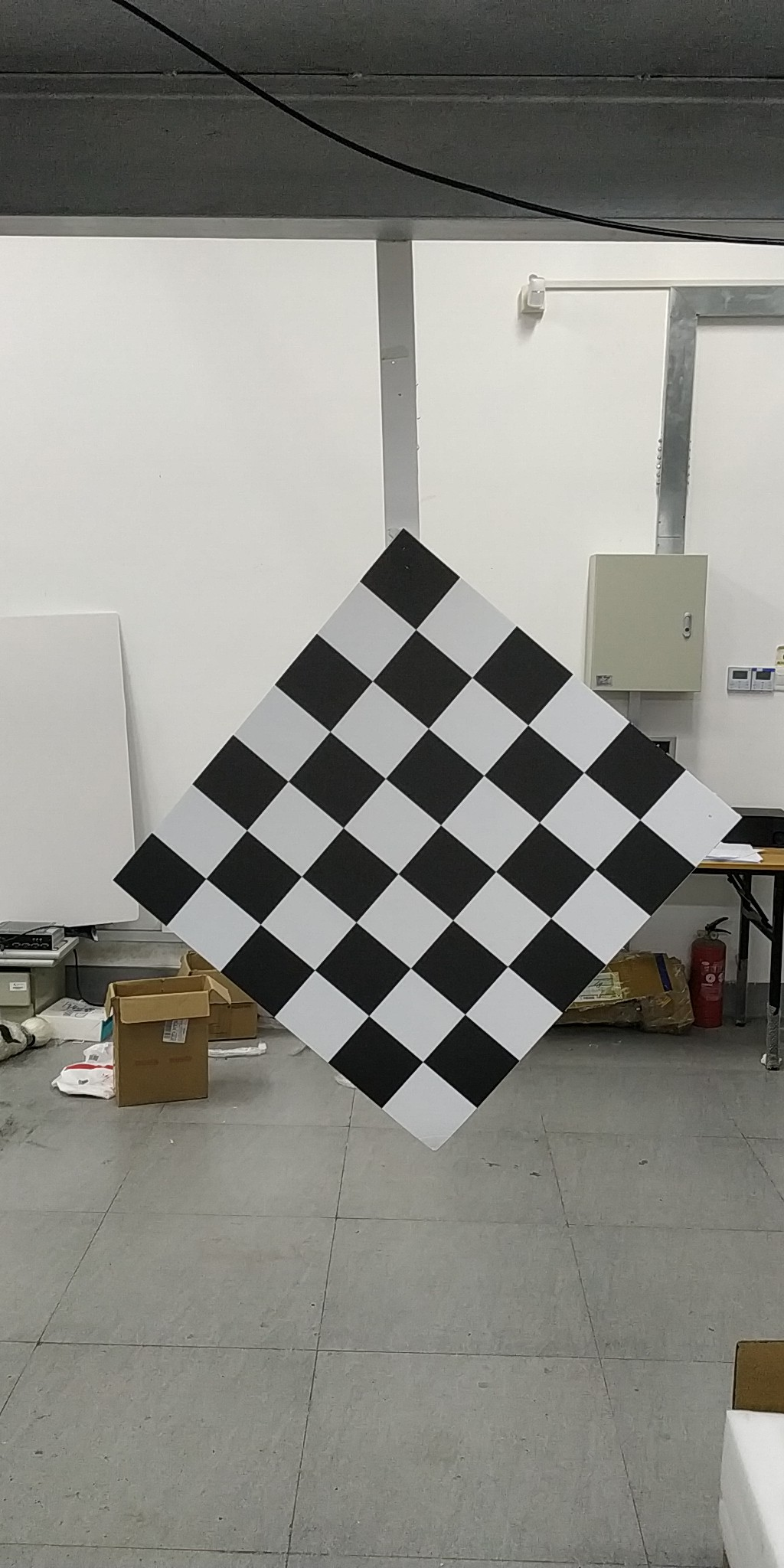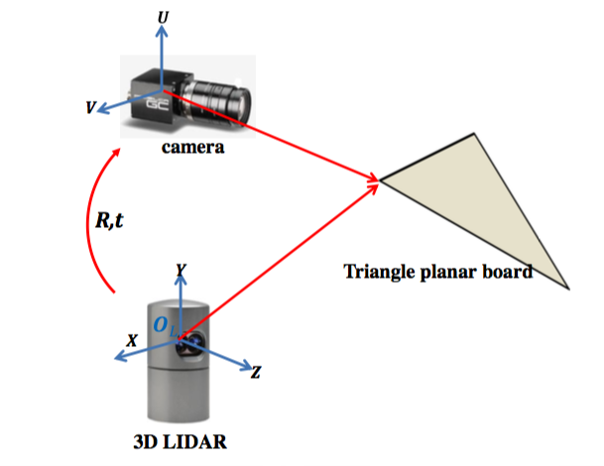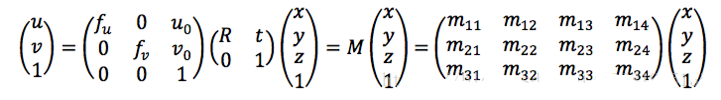# 找寻对应点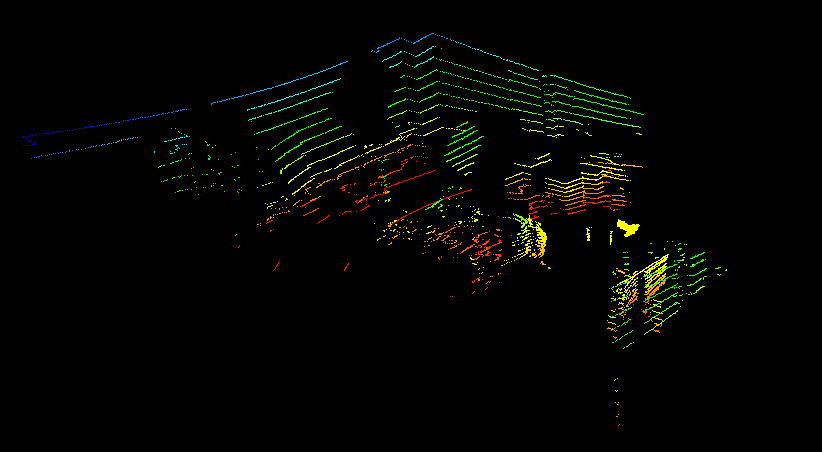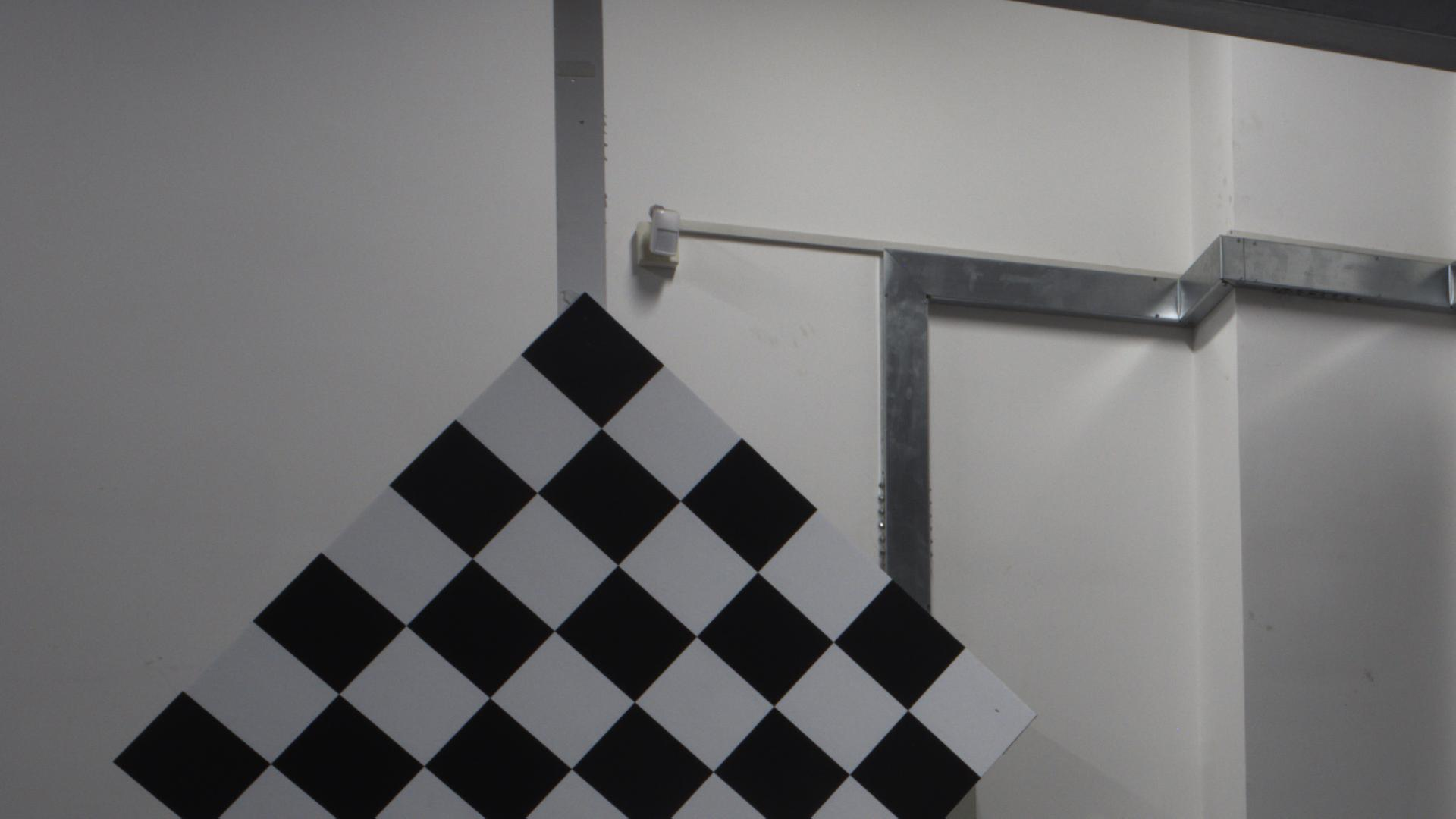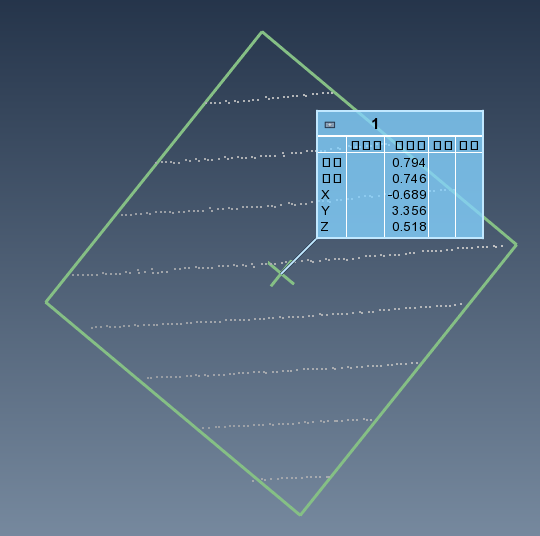# PNP求解

solvePnP(outDim, inDim, cameraMatrix, distCoeff, rvec, tvec);

#include<iostream>
#include<opencv2/opencv.hpp>
#include<vector>
#include<iostream>
#include <string>
#include <vector>
#include <fstream>  //文件流库函数
#include <stdio.h>
#include <stdlib.h>

using namespace std;
using namespace cv;

void SplitString(const string& s, vector<string>& v, const string& c)
{
string::size_type pos1, pos2;
pos2 = s.find(c);
pos1 = 0;
while (string::npos != pos2)
{
v.push_back(s.substr(pos1, pos2 - pos1));

pos1 = pos2 + c.size();
pos2 = s.find(c, pos1);
}
if (pos1 != s.length())
v.push_back(s.substr(pos1));
}

int main()
{

//对应的二维点和三维点对
float threeDim = { { -699, 3349, 1060 }, { -190, 3560, 565 }, { -1190, 3152, 477 }};
float twoDim = { { 771, 385 }, { 1367, 942 }, { 149, 1003 } };

vector<Point3f>outDim;
vector<Point2f>inDim;
vector<float> distCoeff;
distCoeff.push_back(-0.0565);
distCoeff.push_back(0.0643);
distCoeff.push_back(0);
distCoeff.push_back(0);
distCoeff.push_back(0);

for (int i = 0; i < 3; i++)
{
outDim.push_back(Point3f(threeDim[i], threeDim[i], threeDim[i]));
inDim.push_back(Point2f(twoDim[i], twoDim[i]));
}

Mat cameraMatrix(3, 3, CV_32F);

//float tempMatrix = { { 3522.3, 0, 0 }, { 0, 3538.4, 0 }, { 1968.9,1375.4,1.0 } };
float tempMatrix = { { 3522.3, 0, 1968.9 }, { 0, 3538.4, 1375.4 }, { 0, 0, 1.0 } };//相机的内参矩阵

for (int i = 0; i < 3; i++)
{
for (int j = 0; j < 3; j++)
{
cameraMatrix.at<float>(i, j) = tempMatrix[i][j];
}
}

Mat rvec, tvec;
solvePnP(outDim, inDim, cameraMatrix, distCoeff, rvec, tvec);

Rodrigues(rvec, rvec);

//读取txt文件
ifstream infile;
infile.open("C:/Users/18301/Desktop/point.txt");   //将文件流对象与文件连接起来
assert(infile.is_open());   //若失败,则输出错误消息,并终止程序运行

string s;
vector<string> v;
vector<double> tmp;
vector<vector<double>> all_data;
//	all_data.resize(all_data.max_size() + 1);
while (getline(infile, s))
{
//cout << s << endl;
SplitString(s, v, ","); //可按多个字符来分隔;
for (int i = 0; i < 3; i++)
{
tmp.push_back(atof(v[i].c_str())*1000);
}
all_data.push_back(tmp);
v.clear();
tmp.clear();
}

infile.close();             //关闭文件输入流
/*for (int i = 0; i < all_data.size(); i++)
{
cout << all_data[i] << " " << all_data[i] << " " << all_data[i] << endl;
}*/
cout << all_data.size() << endl;
vector<Point3f> all_points;
for (int i = 0; i < all_data.size(); i++)
{
all_points.push_back(Point3f(all_data[i], all_data[i], all_data[i]));
}

std::vector<cv::Point2f> projectedPoints;
cv::projectPoints(all_points, rvec, tvec, cameraMatrix, distCoeff, projectedPoints);
for (int i = 0; i < projectedPoints.size(); i++)
{
Point2f p = projectedPoints[i];
if (p.y<=1080)
{
circle(image, p, 5, Scalar(255, 255, 0), 1, 8, 0);
}

}

imwrite("C:/Users/18301/Desktop/result.jpg", image);
cout << rvec << endl;
cout << tvec << endl;

return 0;

}